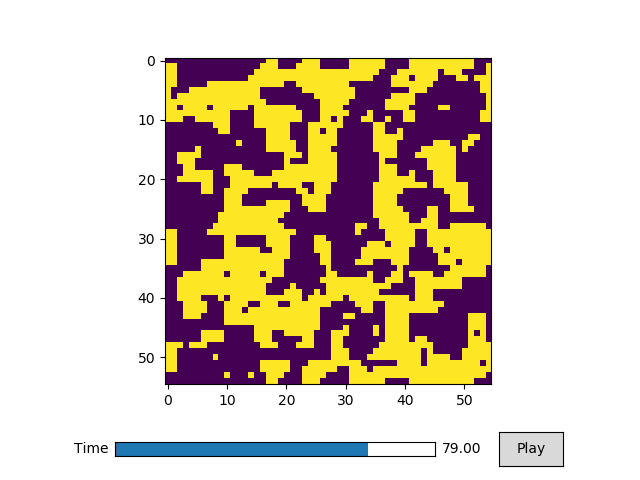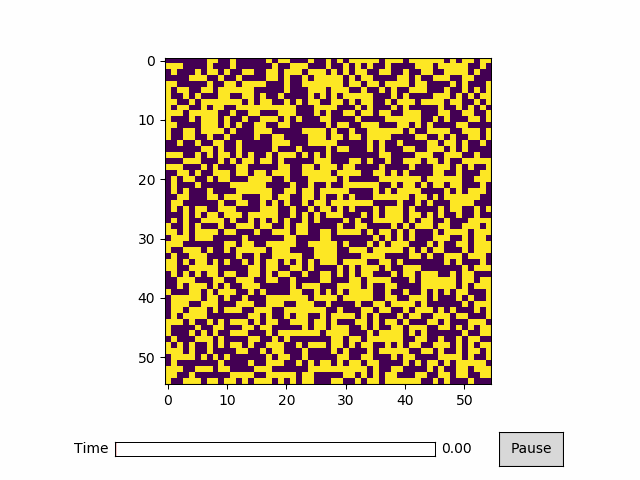# Imshow¶

:

%matplotlib notebook
import numpy as np
import matplotlib.pyplot as plt
import animatplot as amp


First we focus on creating the data. An ising model is used to make this data.

:

# Define LxL matrix
L = 55
# Initialize as random spin
M = 2*(np.random.rand(L,L)>.5)-1
J = 1
b = 2.5

nPer = 100

images = [M]
for i in range(100):
M = M.copy()
for dm in range(nPer):
jj = int(np.random.rand()*L - 1)
kk = int(np.random.rand()*L - 1)
dE = 2*J*(M[jj+1,kk] + M[jj-1,kk] + M[jj,kk+1] + M[jj,kk-1])*M[jj,kk]
if dE <= 0:
M[jj,kk]*=-1
else:
if(np.random.rand()<np.exp(-b*dE)):
M[jj,kk]*=-1
images.append(M)
M[:,-1] = M[:,0]
M[-1,:] = M[0,:]


Now we plot it.

:

block = amp.blocks.Imshow(images)
anim = amp.Animation([block])

anim.controls()
anim.save_gif('ising')
plt.show()